# n-gram模型

P(s) = P(w1, w2, ..., wn) = P(w1) * P(w2|w1) * P(w3|w2,w1) *....*P(wn|wn-1,wn-2,...,w2,w1)


P(S) 被称为语言模型，即用来计算一个句子合法概率的模型。

## 2-gram

P(s) = P(w1, w2, ..., wn) = P(w1) * P(w2|w1) * P(w3|w2) *....*P(wn|wn-1)


## 3-gram

P(s) = P(w1, w2, ..., wn) = P(w1) * P(w2|w1) * P(w3|w2,w1) *....*P(wn|wn-1,wn-2)


# 例子

n-gram 模型通过 计算极大似然估计（Maximum Likelihood Estimate）构造语言模型，这是对训练数据的最佳估计，对于Bigram其计算公式如下：

P(wi|wi-1) = count(wi, wi-1)/count(wi-1)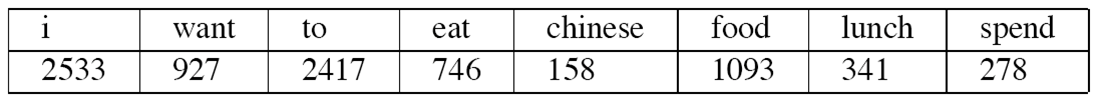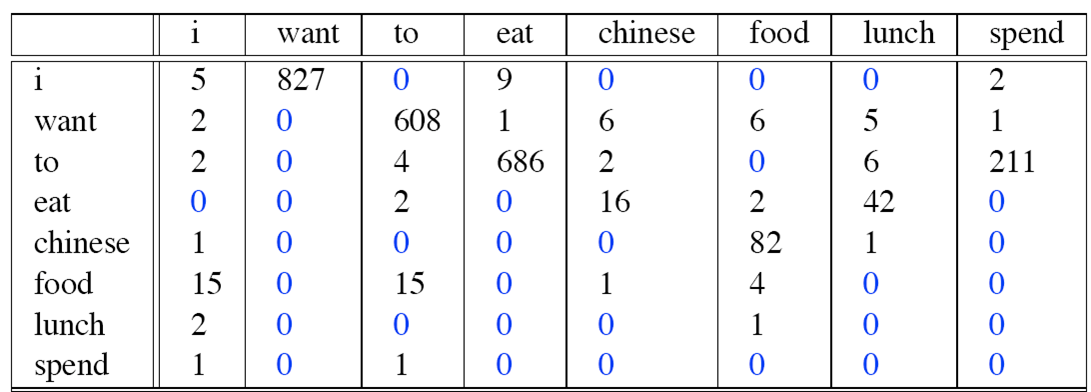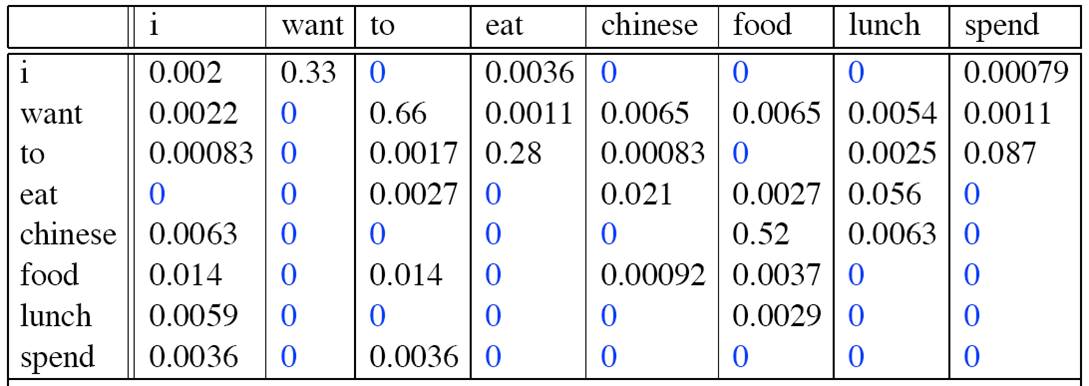P(I want to eat Chinese food) = P(I) * P(want|I) * P(to|want) * P(eat|to) * P(Chinese|eat) * P(food|Chinese)

= （2533/8493) * 0.33 * 0.66 * 0.28 * 0.021 * 0.52。


ps: 取 log 是数据的标准化。

log(p1*p2*p3*p4) = log(p1) + log(p2) + log(p3) + log(p4)


## 用途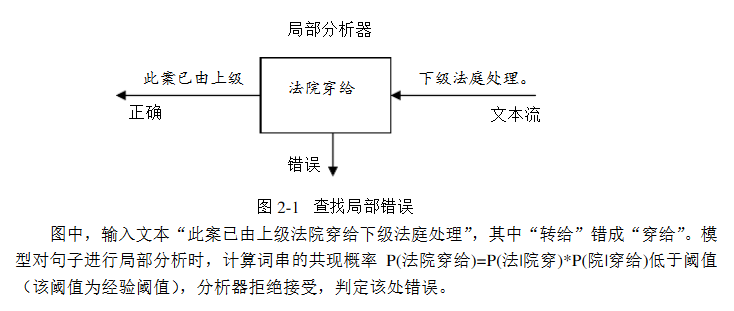# 最短编辑距离

## 编辑距离

sitten （k→s）
sittin （e→i）
sitting （→g）


if （i == 0 且 j == 0），Edit(i, j) = 0
if （i == 0 且 j > 0），Edit(i, j) = j
if （i > 0 且j == 0），Edit(i, j) = i
if （i ≥ 1  且 j ≥ 1） ，Edit(i, j) = min{ Edit(i-1, j) + 1, Edit(i, j-1) + 1, Edit(i-1, j-1) + F(i, j) }，


## 英文实现

import re
from collections import Counter

def words(text): return re.findall(r'\w+', text.lower())

def P(word, N=sum(WORDS.values())):
"Probability of word."
return WORDS[word] / N

def correction(word):
"Most probable spelling correction for word."
return max(candidates(word), key=P)

def candidates(word):
"Generate possible spelling corrections for word."
return (known([word]) or known(edits1(word)) or known(edits2(word)) or [word])

def known(words):
"The subset of words that appear in the dictionary of WORDS."
return set(w for w in words if w in WORDS)

def edits1(word):
"All edits that are one edit away from word."
letters    = 'abcdefghijklmnopqrstuvwxyz'
splits     = [(word[:i], word[i:])    for i in range(len(word) + 1)]
deletes    = [L + R[1:]               for L, R in splits if R]
transposes = [L + R + R + R[2:] for L, R in splits if len(R)>1]
replaces   = [L + c + R[1:]           for L, R in splits if R for c in letters]
inserts    = [L + c + R               for L, R in splits for c in letters]
return set(deletes + transposes + replaces + inserts)

def edits2(word):
"All edits that are two edits away from word."
return (e2 for e1 in edits1(word) for e2 in edits1(e1))


## 工作原理

word –> w correct word –> c

p(c|w)=p(w|c)∗p(c) / p(w)


p(c)为Language Model

 p(w c)为Error Model

c ∈ candidates为Candidate Model

argmax 为 Selection Mechanism

p(c)可从预料库里统计而得，简单的 frequence / sum_number

 p(c w) 需要 data on spelling errors

### 改进

correction(‘addresable’) => ‘addresable’ (0); expected ‘addressable’ (0)


### 对于p(w|c)

correction(‘adres’) => ‘acres’ (37); expected ‘address’ (77)


（1）同音字/谐音字

（2）形近字

（3）同义词

（4）字词乱序、字词增删

## 美团的实现方式

• 支持前缀匹配原则

• 同时支持汉字、拼音输入

• 支持多音字输入提示

• 支持拼音缩写输入

• 基于用户的历史搜索行为，按照关键字热度进行排序

# 中文语法纠错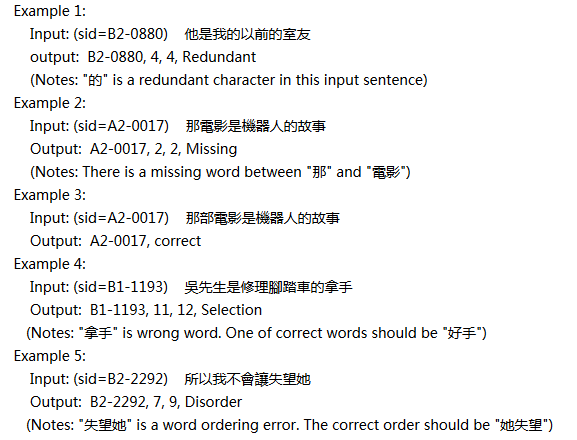## 依存树

【论文截图】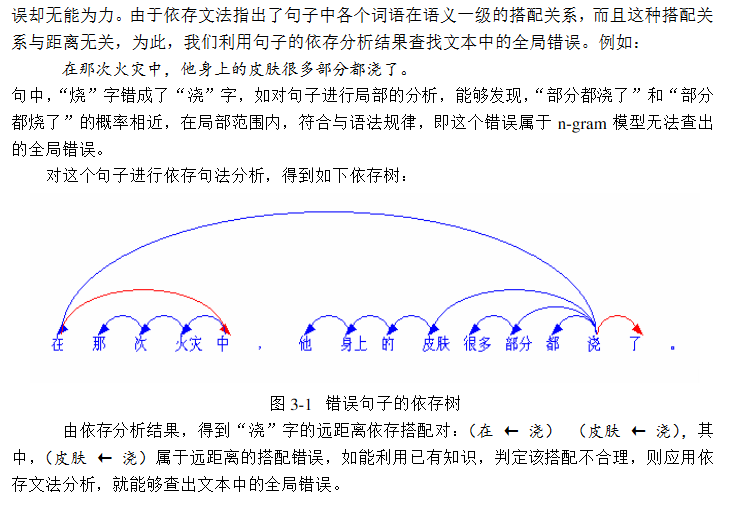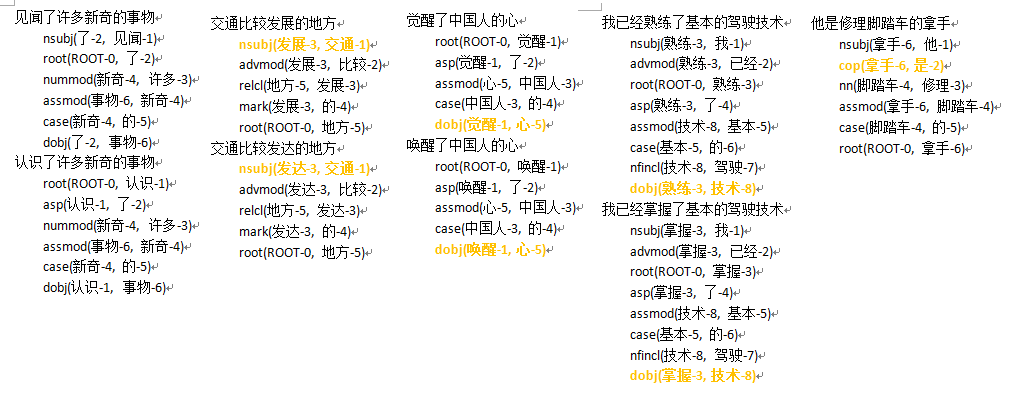• 同义词

# 依存树同义词查找

ESA的主要思想就是，将一个Wiki词条看成一个主题概念，然后将词条下的解释文本先用TF-IDF逆文档频率过滤分词，再用倒排索引建立成（word-Topic），这样就可以构造主题向量，我们可以用这些主题向量来做语义相似度计算，完成同义词的查找。

ps: 这种方法工作量巨大，需要特别大的样本，基本词库可以选取一些整理好的的同义词词库。

# N-Gram 的数据来源

## 分词计算 n-gram 的方式

#coding=utf-8
'''
Created on 2018-2-6

'''
# 这里的_word_ngrams方法其实就是sklearn中CountVectorizer函数中用于N-Gram的方法，位置在D:\Python27\Lib\site-packages\sklearn\feature_extraction\text.py

def _word_ngrams(tokens, stop_words=None,ngram_range=(1,1)):
"""Turn tokens into a sequence of n-grams after stop words filtering"""
# handle stop words
if stop_words is not None:
tokens = [w for w in tokens if w not in stop_words]

# handle token n-grams
min_n, max_n = ngram_range
if max_n != 1:
original_tokens = tokens
tokens = []
n_original_tokens = len(original_tokens)
for n in xrange(min_n,
min(max_n + 1, n_original_tokens + 1)):
for i in xrange(n_original_tokens - n + 1):
tokens.append(" ".join(original_tokens[i: i + n]))


• 测试代码
text = "我去云南旅游，不仅去了玉龙雪山，还去丽江古城，很喜欢丽江古城"
import jieba
cut = jieba.cut(text)
listcut = list(cut)
n_gramWords = _word_ngrams(tokens = listcut,ngram_range=(2,2))
print n_gramWords
for n_gramWord in n_gramWords:
print n_gramWord
# 我 去
# 去 云南旅游
# 云南旅游 ，
# ， 不仅
# 不仅 去
# 去 了
# 了 玉龙雪山
# 玉龙雪山 ，
# ， 还
# 还 去
# 去 丽江
# 丽江 古城
# 古城 ，
# ， 很
# 很 喜欢
# 喜欢 丽江
# 丽江 古城


# 基于n-gram模型的中文分词

## 最大化概率2-gram分词算法

1、将带分词的字符串从左到右切分为w1,w2,…,wi；

2、计算当前词与所有前驱词的概率

3、计算该词的累计概率值，并筛选最大的累计概率则为最好的前驱点；

4、重复步骤3，直到该字符串结束；

5、从wi开始，按照从右到左的顺序，依次将没歌词的最佳前驱词输出，即字符串的分词结束。

## 算法框架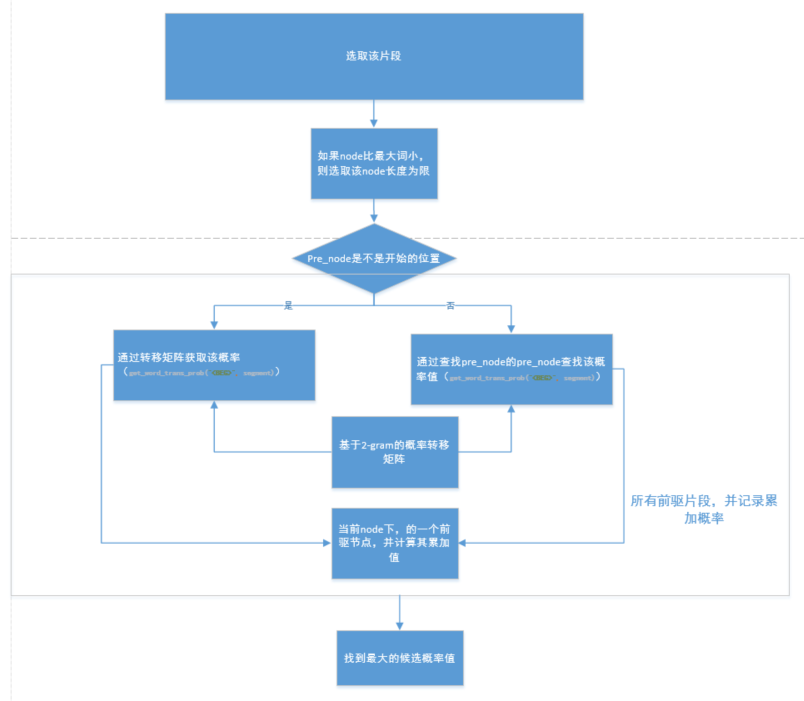word_dict = {}# 用于统计词语的频次
transdict = {} # 用于统计该词后面词出现的个数
def train(train_data_path):
transdict['<BEG>'] = {}#<beg>表示开始的标识
word_dict['<BEG>'] = 0
for sent in open(train_data_path,encoding='utf-8'):
word_dict['<BEG>'] +=1
sent = sent.strip().split(' ')
sent_list = []
for word in sent:
if word !='':
sent_list.append(word)
for i,word in enumerate(sent_list):
if word not in word_dict:
word_dict[word] = 1
else:
word_dict[word] +=1
# 统计transdict bi-gram<word1,word2>
word1,word2 = '',''
# 如果是句首，则为<beg,word>
if i == 0:
word1,word2 = '<BEG>',word
# 如果是句尾，则为<word,END>
elif i == len(sent_list)-1:
word1,word2 = word,'<END>'
else:
word1,word2 = word,sent_list[i+1]
# 统计当前次后接词出现的次数
if word not in transdict.keys():
transdict[word1]={}
if word2 not in transdict[word1]:
transdict[word1][word2] =1
else:
transdict[word1][word2] +=1
return word_dict,transdict

# 最大化概率2-gram分词
import math
word_dict = {}# 统计词频的概率
trans_dict = {}# 当前词后接词的概率
trans_dict_count = {}#记录转移词频
max_wordLength = 0# 词的最大长度
all_freq = 0 # 所有词的词频总和
train_data_path = "D:\workspace\project\\NLPcase\\ngram\\data\\train.txt"
from ngram import ngramTrain
word_dict_count,Trans_dict = ngramTrain.train(train_data_path)
all_freq = sum(word_dict_count)
max_wordLength = max([len(word) for word in word_dict_count.keys()])
for key in word_dict_count:
word_dict[key] = math.log(word_dict_count[key]/all_freq)
# 计算转移概率
for pre_word,post_info in Trans_dict.items():
for post_word,count in post_info:
word_pair = pre_word+' '+post_word
trans_dict_count[word_pair] = float(count)
if pre_word in word_dict_count.keys():
trans_dict[word_pair] = math.log(count/word_dict_count[pre_word])
else:
trans_dict[word_pair] = word_dict[post_word]

# 估算未出现词的概率，平滑算法
def get_unk_word_prob(word):
return math.log(1.0/all_freq**len(word))
# 获取候选词的概率
def get_word_prob(word):
if word in word_dict:
prob = word_dict[word]
else:
prob = get_unk_word_prob(word)
return prob
# 获取转移概率
def get_word_trans_prob(pre_word,post_word):
trans_word = pre_word+" "+post_word
if trans_word in trans_dict:
trans_prob = math.log(trans_dict_count[trans_word]/word_dict_count[pre_word])
else:
trans_prob = get_word_prob(post_word)
return trans_prob
# 寻找node的最佳前驱节点，方法为寻找所有可能的前驱片段
def get_best_pre_nodes(sent,node,node_state_list):
# 如果node比最大词小，则取的片段长度的长度为限
max_seg_length = min([node,max_wordLength])
pre_node_list = []# 前驱节点列表

# 获得所有的前驱片段，并记录累加概率
for segment_length in range(1,max_seg_length+1):
segment_start_node = node - segment_length
segment = sent[segment_start_node:node]# 获取前驱片段
pre_node = segment_start_node# 记录对应的前驱节点
if pre_node == 0:
# 如果前驱片段开始节点是序列的开始节点，则概率为<S>转移到当前的概率
segment_prob = get_word_trans_prob("<BEG>",segment)
else:# 如果不是序列的开始节点，则按照二元概率计算
# 获得前驱片段的一个词
pre_pre_node = node_state_list[pre_node]["pre_node"]
pre_pre_word = sent[pre_pre_node:pre_node]
segment_prob = get_word_trans_prob(pre_pre_word,segment)
pre_node_prob_sum = node_state_list[pre_node]["prob_sum"]
# 当前node一个候选的累加概率值
candidate_prob_sum = pre_node_prob_sum+segment_prob
pre_node_list.append((pre_node,candidate_prob_sum))
# 找到最大的候选概率值
(best_pre_node, best_prob_sum) = max(pre_node_list,key=lambda d:d)
return best_pre_node,best_prob_sum
def cut(sent):
sent = sent.strip()
# 初始化
node_state_list = []#主要是记录节点的最佳前驱，以及概率值总和
ini_state = {}
ini_state['pre_node'] = -1
ini_state['prob_sum'] = 0 #当前概率总和
node_state_list.append(ini_state)
# 逐个节点的寻找最佳的前驱点
for node in range(1,len(sent)+1):
# 寻找最佳前驱，并记录当前最大的概率累加值
(best_pre_node,best_prob_sum) = get_best_pre_nodes(sent,node,node_state_list)
# 添加到队列
cur_node ={}
cur_node['pre_node'] = best_pre_node
cur_node['prob_sum'] = best_prob_sum
node_state_list.append(cur_node)
# 获得最优路径，从后到前
best_path = []
node = len(sent)
best_path.append(node)
while True:
pre_node = node_state_list[node]['pre_node']
if pre_node ==-1:
break
node = pre_node
best_path.append(node)
# 构建词的切分
word_list = []
for i in range(len(best_path)-1):
left = best_path[i]
right = best_path[i+1]

word = sent[left:right]
word_list.append(word)
return word_list


## 基于ngram的前向后向最大匹配算法

### 算法描述

1、利用最大向前和向后的算法对待句子进行切分，分别得到两个字符串s1和s2

2、如果得到两个不同的词序列，则根据bi-gram选择概率最大的（此方法可以消除歧义）；

3、计算基于bi-garm的句子生成概率；

### 代码

# 最要是基于bi-gram的最大前向后向算法，对中文进行分词

import math
from ngram import ngramTrain
from maxMatch import maxBackforward
from maxMatch import maxForward
train_data_path = "D:\workspace\project\\NLPcase\\ngram\\data\\train.txt"
word_counts = 0# 语料库中的总词数
word_types = 0#语料库中的词种类数目
word_dict,trans_dict = ngramTrain.train(train_data_path)
word_types = len(word_dict)
word_counts = sum(word_dict.values())

# 计算基于ngram的句子生成概率
def compute_likehood(seg_list):
p = 0
# 由于概率很小，对连乘取对数转化为加法
for pos,words in enumerate(seg_list):
if pos < len(seg_list)-1:
# 乘以后面的条件概率
word1,word2 = words,seg_list[pos+1]
if word1 not in trans_dict:
# 加平滑，让个该词至少出现1次
p += math.log(1.0/word_counts)
else:
# 加1平滑
fenzi, fenmu = 1.0,word_counts
# 计算转移概率，如p(w1/w2) 为在转移矩阵中trans_dict[word1][word2]在整行的占比
for key in trans_dict[word1]:
if key == word2:
fenzi +=trans_dict[word1][word2]
fenmu += trans_dict[word1][key]
# log(p(w0)*p(w1|w0)*p(w2|w1)*p(w3|w2)) == log(w0)+ log(p(w1|w0))+ log(p(w2|w1)) + log(p(w3|w2))
p +=math.log(fenzi/fenmu)
# 乘以第一个词的概率
if (pos == 0 and words !='<BEG>') or (pos == 1 and seg_list == '<BEG>'):
if words in word_dict:
p += math.log((float(word_dict[words])+1.0)/word_types+word_counts)
else:
# 加1进行平滑
p +=math.log(1.0/(word_types+word_counts))
return p
# 然后进行分词
def cut_main(sent):
seg_list1 = maxForward.maxForwardCut(sent,5,word_dict)
seg_list = []
# differ_list1和differ_list2分布及记录两个句子词序不同的部分，用于消除歧义
differ_list1 = []
differ_list2 = []
# pos1和pos2记录两个句子的当前字的位置，cur1和cur2记录两个句子的第几个词
pos1 = pos2 = 0
cur1=cur2 = 0
while 1:
if cur1 == len(seg_list1) and cur2 == len(seg_list2):
break
if pos1 == pos2:# 位置相同

if len(seg_list1[cur1]) == len(seg_list2[cur2]):# 对应的词的长度也相同
pos1 +=len(seg_list1[cur1])# 移到下一位置
pos2 += len(seg_list2[cur2]) # 移到下一位置
if len(differ_list1)>0:
# 说明此时得到两个不同的词序列，根据bi-gram选择概率大的
# 注意不同的时候哟啊考虑加上前面一个词和后面一个词，拼接的时候在去掉
differ_list1.insert(0,seg_list[-1])
differ_list2.insert(0,seg_list[-1])
if cur1 < len(seg_list1)-1:
differ_list1.append(seg_list1[cur1])
differ_list2.append(seg_list2[cur2])
p1 = compute_likehood(differ_list1)
p2 = compute_likehood(differ_list2)
if p1>p2:
differ_list = differ_list1
else:
differ_list = differ_list2
differ_list.remove(differ_list)
if cur1<len(seg_list1)-1:
differ_list.remove(seg_list1[cur1])
for words in differ_list:
seg_list.append(words)
differ_list1 = []
differ_list2 = []
seg_list.append(seg_list1[cur1])
cur1 +=1
cur2 +=1
elif len(seg_list1[cur1]) > len(seg_list2[cur2]):
differ_list2.append(seg_list2[cur2])# 记录的其实是前一个词
pos2 += len(seg_list2[cur2])
cur2 +=1
else:
differ_list1.append(seg_list1[cur1])# 记录的其实是前一个词
pos1 +=len(seg_list1[cur1])
cur1 +=1
else:
if pos1 + len(seg_list1[cur1]) == pos2 + len(seg_list2[cur2]):
differ_list1.append(seg_list1[cur1])
differ_list2.append(seg_list2[cur2])
pos1 += len(seg_list1[cur1])
pos2 += len(seg_list2[cur2])
cur1 += 1
cur2 += 1

elif pos1+len(seg_list1[cur1])>pos2+len(seg_list2[cur2]):
differ_list2.append(seg_list2[cur2])
pos2 += len(seg_list2[cur2])
cur2 += 1
else:
differ_list1.append(seg_list1[cur1])
pos1 += len(seg_list1[cur1])
cur1 += 1
return seg_list


# 源码参考

word-checker 中文英文纠错

pinyin 拼音转换

segment 分词框架